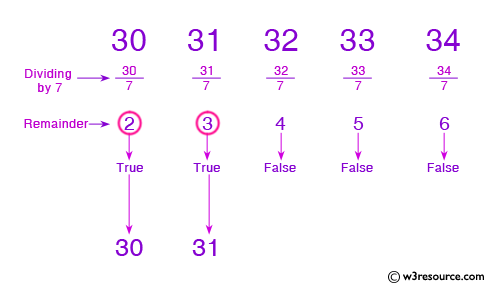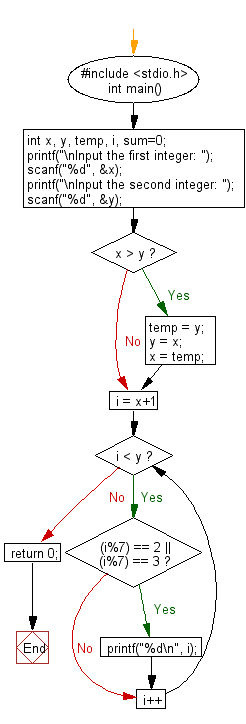﻿ C : Find numbers that divide by 7 with a remainder of 2 or 3

# C Exercises: Find all numbers which are dividing by 7 and the remainder is equal to 2 or 3 between two given integer numbers

## C Basic Declarations and Expressions: Exercise-40 with Solution

Write a C program that finds all integer numbers that divide by 7 and have a remainder of 2 or 3 between two given integers.Sample Solution:

C Code:

``````#include <stdio.h>
int main() {
int x, y, temp, i, sum=0;

// Prompt for user input
printf("\nInput the first integer: ");
scanf("%d", &x);
printf("\nInput the second integer: ");
scanf("%d", &y);

// Swap values if x is greater than y
if(x > y) {
temp = y;
y = x;
x = temp;
}

// Iterate through numbers between x and y (exclusive)
for(i = x+1; i < y; i++) {
// Check if the number modulo 7 is 2 or 3
if((i%7) == 2 || (i%7) == 3) {
printf("%d\n", i);
}
}

return 0;
}
``````

Sample Output:

```Input the first integer: 25

Input the second integer: 45
30
31
37
38
44
```

Flowchart:C programming Code Editor:

What is the difficulty level of this exercise?

Test your Programming skills with w3resource's quiz.

﻿